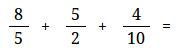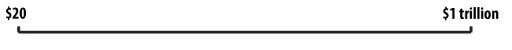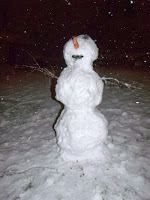Posts

Showing posts from December, 2013

Open vs. closed math problems

As you are gearing toward the new year, I want you to watch this short video by Jo Boaler. Seriously. Take a few minutes. It's not long, and I think you will be glad you did!

Jo mentioned the simple task of finding the perimeter of a rectangle when its sides are given, and changing that into a problem where students are asked to give two different rectangles with a given perimeter.

That is changing the task from a "closed" format, or a simple performance task, into an "open" format and into a LEARNING/GROWING type of task. It essentially means that now the problem has many possible answers, instead of just one. And it was a very simple change that did it!

Then... read my article From CLOSED math problems (with one answer) to OPEN ones

After reading... can you find a closed problem you can change and "open up"? Share it!

Fraction worksheet generator updatedI know this doesn't interest everyone... : ) It has to do with the fraction worksheet generator at HomeschoolMath.net.  I have worked on it in preparation for the release of the new version of Math Mammoth grade 6.

A new feature is that you can now make problems with 3 or 4 fractions, like this:

(Add and subtract 3 unlike fractions)

or multiplications like this:

(Multiply four fractions worksheet)

Then, you can use negative fractions (think pre-algebra / algebra 1) and practice addition:

or subtraction:

(or multiplication or division).

Try them here: Add or subtract negative fractions worksheet

Another big change is that now you can choose addition/subtraction (instead of just one operation), which then generates both addition and subtraction problems on the same sheet, and in the case of 3 or 4 fractions, includes both addition and subtraction in the problem.

Of course, you can generate regular addition/subtraction/multiplication/division problems with fractions and/or mixed numb…

Can you place 1 million on a number line?Denise wrote something interesting  relating to very LARGE NUMBERS, sort of a misconception that we all seem to have.

She asked students to mark 1 million on a number line that went from \$20 to \$1 billion. Here's the number line. Can you do it?

Give it an honest try!

Then go read how her students fared and see the right answer... you might be surprised!

She said, "Our intuitive number sense seems to work like a semi-logarithmic scale, with the small numbers evenly spaced and the bigger numbers crowded together at the end."

Free worksheets for area of triangles, quadrilaterals, and other polygonsOK, maybe this is not the best time of year to mention this (I realize your minds may be on other things :), but if I don't do it now, I may forget it later : (

I have made a new worksheet generator... for areas of triangles, parallelograms, trapezoids, generic quadrilaterals, pentagons, and/or hexagons (you can choose the shape(s) to be used).

It uses the coordinate grid. You can select either the first quadrant or all quadrants.

http://www.homeschoolmath.net/worksheets/area_triangles_polygons.php

Something like this:

The answer key shows the polygon drawn in the coordinate grid, and of course the area in square units.

This is a focus topic typically for 6th grade math, but may also appear in 5th and 7th grades.

Snowman math!Ready for some snowman math? I checked around on the web and found some neat activities for your little ones.

Making Learning Fun has many snowman activities and printables for preschool and kindergarten folks, such as counting, color by number, or simple addition.
MathWire has a whole page dedicated to snowman math activities, for about K-2nd grade level.

Check for example the estimation activity on that page, or count by fives printable. Another good one is snowman combinations - children figure out how many different kinds of snowmen can be built with a certain number of possible 'parts'.

Then, here's a snowman puzzle from Jigzone. You can solve it right here on my blog with a 20-piece cut, but if you go solve it at Jigzone, you can change the cut so it has more or less pieces.

Jigzone also has another, a snowman photo puzzle.

Finally, this is not math, but it is a neat story for little ones you can read for free (online), and beautifully illustrated: Snowmen at Nig…

Currclick Cyber Week saleCurrclick is having Cyber Monday - or actually Cyber Week - sale.

Lots of deals, including you can get Math Mammoth products at 20% off.

Enjoy!